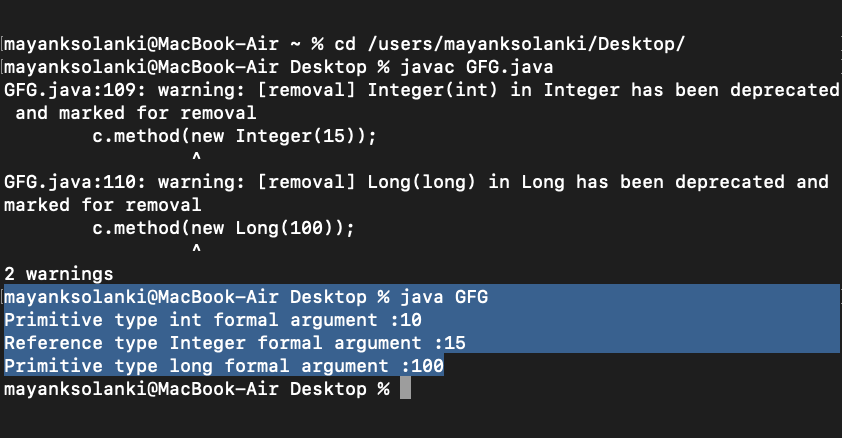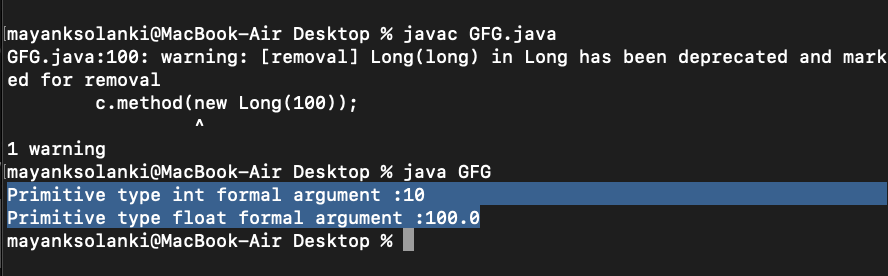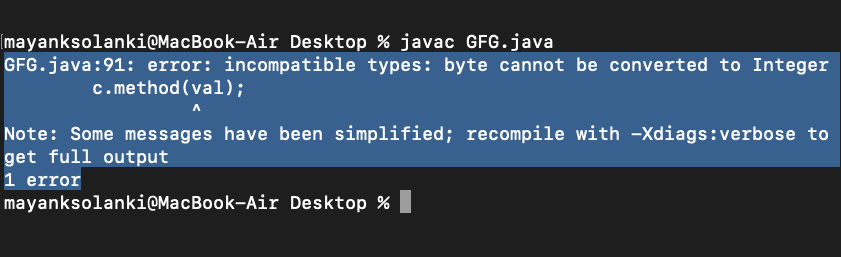GFG App
Open AppBrowser
Continue

Let us go through the basic pre-requisites such as Method Overloading, Autoboxing, and Unboxing. So method overloading in java is based on the number and type of the parameters passed as an argument to the methods. We can not define more than one method with the same name, Order, and type of the arguments. It would be a compiler error. The compiler does not consider the return type while differentiating the overloaded method. But you cannot declare two methods with the same signature and different return types. It will throw a compile-time error. If both methods have the same parameter types, but different return types, then it is not possible.

Autoboxing is the process of converting a primitive value into an object of the corresponding wrapper class is called autoboxing. For example, converting int to Integer class. While Unboxing is a process of converting an object of a wrapper type to its corresponding primitive value is called unboxing. For example conversion of Integer to int.

In Java, there are two types of variables:

• Primitive type
• Reference type.

Conversion of primitive type to its corresponding wrapper Object is called Autoboxing and Wrapper Object to its corresponding primitive type is known as Unboxing.

In method overloading, you may come across a situation where a signature takes reference type or a primitive type as a formal argument. The compiler first searches a method with parameter(s) of the same data type(s). If you are using wrapper class Object as an actual argument and the compiler does not find the method with parameter(s) of the same reference type (i.e. class or interface type), then it starts searching a method with parameter(s) having the corresponding primitive data type.

Example

## Java

 `// Java Program to Illustrate Autoboxing` `// While resolving data type as:` `// (a) reference` `// (b) primitive`   `// Importing required classes` `import` `java.io.*;`   `// Class 1` `// Helper class` `class` `Conversion {`   `    ``// Method 1` `    ``// Overloading method with primitive formal argument` `    ``public` `void` `method(``int` `i)` `    ``{`   `        ``// Print statement` `        ``System.out.println(` `            ``"Primitive type int formal argument :"` `+ i);` `    ``}`   `    ``// Method 2` `    ``// Overloading method with reference formal argument` `    ``public` `void` `method(Integer i)` `    ``{`   `        ``// Print statement` `        ``System.out.println(` `            ``"Reference type Integer formal argument :"` `+ i);` `    ``}`   `    ``// Method 2` `    ``// Overloading method primitive formal argument` `    ``// and to be invoked for wrapper Object as overloaded` `    ``// method with wrapper object of same(Long) type as an` `    ``// argument is not available.` `    ``public` `void` `method(``long` `i)` `    ``{`   `        ``// Print statement` `        ``System.out.println(` `            ``"Primitive type long formal argument :"` `+ i);` `    ``}` `}`   `// Class 2` `// Main class` `class` `GFG {`   `    ``// main driver method` `    ``public` `static` `void` `main(String[] args)` `    ``{`   `        ``// Creating instance of class 1 inside main() method` `        ``Conversion c = ``new` `Conversion();`   `        ``// Invoking the method with different signature` `        ``c.method(``10``);` `        ``c.method(``new` `Integer(``15``));` `        ``c.method(``new` `Long(``100``));`   `        ``// Using short will give, argument mismatch;` `        ``// possible lossy conversion from int to short` `        ``// c.method(new Short(15));` `    ``}` `}`

Output:If the compiler fails to find any method corresponding to autoboxing, then it starts searching a method parameter(s) of the widened primitive data type.

Implementation:

In the example below, we are invoking the overloaded method with primitive(int) formal argument that has the same data type as the actual argument’s data type. We are invoking another method with the argument of Long wrapper Object. The compiler starts searching for the method having the same reference type (Long wrapper class). Since there is no method having with parameter of Long wrapper class. So, It searches for a method that can accept the parameter bigger than long primitive data type as an argument. In this case, it finds a method with float primitive data type and invokes it.

Example

## Java

 `// Java Program to Illustrate method Overloading` `// In case of Widening`   `// Importing required classes` `import` `java.io.*;`   `// Class 1` `// Helper class` `class` `Conversion {`   `    ``// Method` `    ``// overloaded method` `    ``public` `void` `method(``int` `i) {`   `        ``// Print statement` `        ``System.out.println(` `            ``"Primitive type int formal argument :"` `+ i);` `    ``}`   `    ``// Method 2` `    ``// overloaded method primitive formal argument` `    ``// and to be invoked for wrapper Object as` `    ``public` `void` `method(``float` `i) {`   `        ``// Print statement` `        ``System.out.println(` `            ``"Primitive type float formal argument :"` `+ i);` `    ``}` `}`   `// Class 2` `// Main class` `class` `GFG {`   `    ``// Main driver method` `    ``public` `static` `void` `main(String[] args) {`   `        ``// Creating instance of class 1` `        ``Conversion c = ``new` `Conversion();`   `        ``// Invoking(calling) method with signature` `        ``// has widened data type` `        ``c.method(``10``);` `        ``c.method(``new` `Long(``100``));` `    ``}` `}`

Output:Note:

• Priority Order for Primitive types: Same type > Auto Widening > Boxing > Upcasting(Parent Class) > Super Class
• Priority Order for Reference types: Same type > Upcasting(Parent Class) > Super Class > Unboxing

Geeks, ever wondered what happens if widening and boxing happen together? What method of invocation will the compiler be able to do?  Widening of primitive types has taken priority over boxing and var-args. But widening and boxing of primitive types can not work together.

Example 1A

## Java

 `// Java program  to illustrate Method Overloading for` `// Widening and autoboxing Together`   `// Importing required classes` `import` `java.io.*;`   `// Class 1` `// Helper class` `public` `class` `Conversion {`   `    ``// Method` `    ``// Overloading method with` `    ``// reference type formal argument` `    ``public` `void` `method(Integer a)` `    ``{`   `        ``// Print statement` `        ``System.out.println(` `            ``"Primitive type byte formal argument :"` `+ a);` `    ``}` `}`   `// Class 2` `// Main class` `class` `GFG {`   `    ``// Main driver method` `    ``public` `static` `void` `main(String[] args)` `    ``{`   `        ``// Creating an object of above class` `        ``Conversion c = ``new` `Conversion();`   `        ``// Calling method defined in above class` `        ``// inside main() method` `        ``byte` `val = ``5``;` `        ``c.method(val);` `    ``}` `}`

Output:Note: Geeks, but boxing followed by Upcasting is acceptable if this is passed to a reference of type Object for which we will be proposing another example as follows:

Example 1B

## Java

 `// Java program to illustrate Autoboxing Followed by` `// Widening in Reference Type Variables`   `// Importing required classes` `import` `java.io.*;`   `// Class 1` `// helper class` `public` `class` `Conversion {`   `    ``// Method` `    ``// Overloading method with reference type` `    ``// formal argument` `    ``public` `void` `method(Object b)` `    ``{`   `        ``// Object b is typecasted to Byte and then printed` `        ``Byte bt = (Byte)b;`   `        ``// Print statement` `        ``System.out.println(` `            ``"reference type formal argument :"` `+ bt);` `    ``}` `}`   `// Class 2` `// Main class` `class` `GFG {`   `    ``// Main driver method` `    ``public` `static` `void` `main(String[] args)` `    ``{`   `        ``// Creating an instance of class 1 inside main()` `        ``// method` `        ``Conversion c = ``new` `Conversion();`   `        ``byte` `val = ``5``;`   `        ``// b is first widened to Byte` `        ``// and then Byte is passed to Object` `        ``c.method(val);` `    ``}` `}`

Output

`reference type formal argument :5`

Note: Widening of primitive type gets more priority over var-args.

Example

## Java

 `// Java program to illustrate Method Overloading` `// for var-args and Widening concept Together`   `// Importing required classes` `import` `java.io.*;`   `// Class 1` `// Helper class` `public` `class` `Conversion {`   `    ``// Overloading method primitive(byte) var-args formal` `    ``// argument` `    ``public` `void` `method(``byte``... a)` `    ``{`   `        ``// Print statement` `        ``System.out.println(` `            ``"Primitive type byte formal argument :"` `+ a);` `    ``}`   `    ``// Method 2` `    ``// Overloading method primitive(int) formal arguments` `    ``public` `void` `method(``long` `a, ``long` `b)` `    ``{`   `        ``// Print statement` `        ``System.out.println(` `            ``"Widening type long formal argument :"` `+ a);` `    ``}` `}`   `// Class 2` `// Main class` `class` `GFG {`   `    ``// Main driver method` `    ``public` `static` `void` `main(String[] args)` `    ``{`   `        ``// Creating an instance of class 1 inside main()` `        ``// method` `        ``Conversion c = ``new` `Conversion();`   `        ``// Invokes the method having widening` `        ``// primitive type parameters` `        ``byte` `val = ``5``;` `        ``c.method(val, val);` `    ``}` `}`

Output

`Widening type long formal argument :5`

This article is contributed by Nitsdheerendra. If you like GeeksforGeeks and would like to contribute, you can also write an article using write.geeksforgeeks.org or mail your article to review-team@geeksforgeeks.org. See your article appearing on the GeeksforGeeks main page and help other Geeks.